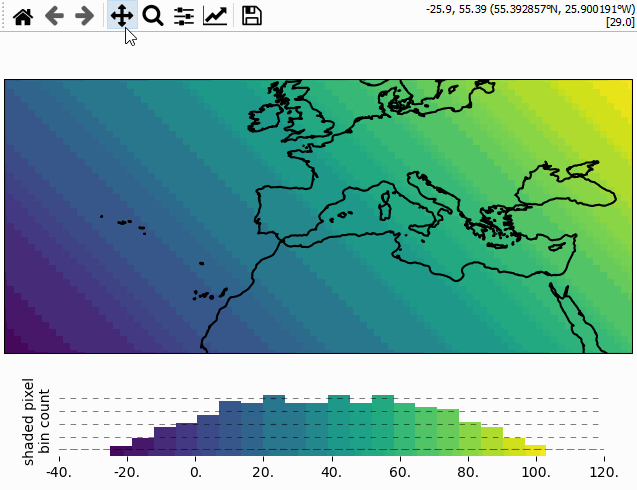# 🔴 Data Visualization

## Quick overview

To visualize a dataset, first assign the dataset to the `Maps` object, then select how you want to visualize the data and finally call `Maps.plot_map()`.

1. Assign the data to a `Maps` object via `Maps.set_data()`

2. (optional) Set the shape used to represent the data via `Maps.set_shape`

3. (optional) Classify the data via `Maps.set_classify`

4. Plot the data by calling `Maps.plot_map()`

```from eomaps import Maps
m = Maps()

m_data = m.new_layer()                         # Create a new layer for the data
m_data.set_data(                               # Assign the data
data=[1, 2, 3, 4, 5],                      #    Data values
x=[-10, 20, 30, 40, 50],                   #    x coordinate values
y=[-50, -20, 0, 20, 40],                   #    y coordinate values
crs=4326                                   #    Coordinate system of (x, y)
)

m_data.set_classify.EqualInterval(k=3)         # Classify into 3 equal intervals
m_data.plot_map(vmin=0, ec="k", cmap="magma")  # Plot the data
```

Note

A `Maps` object can only manage a single dataset!

To plot multiple datasets on the same map, use `Maps.new_layer()` to get a unique `Maps` object for each dataset! To quickly create a new layer that uses the same dataset, cassification and shape as the parent, use:

```m_1 = m.new_layer(inherit_data=True,            # m_1 inherits the data from m
inherit_classification=True,  # m_1 inherits the classification and colormap from m
inherit_shape=True            # m_1 inherits the plot-shape from m
)
```

## 1) Assign the data

To assign a dataset to a `Maps` object, use `Maps.set_data()`.

 `Maps.set_data` Set the properties of the dataset you want to plot.

A dataset is fully specified by setting the following properties:

• `data` : The data-values

• `x`, `y`: The coordinates of the provided data

• `crs`: The coordinate-reference-system of the provided coordinates

• `parameter` (optional): The parameter name

• `encoding` (optional): The encoding of the data

• `cpos`, `cpos_radius` (optional): the pixel offset

Note

Make sure to use a individual `Maps` object (e.g. with `m2 = m.new_layer()` for each dataset! Calling `Maps.plot_map()` multiple times on the same `Maps` object will remove and override the previously plotted dataset!

A note on data-reprojection…

EOmaps handles the reprojection of the data from the input-crs to the plot-crs.

• Plotting data in its native crs will omit the reprojection step and is therefore a lot faster!

• If your dataset is 2D (e.g. a raster), it is best (for speed and memory) to provide the coordinates as 1D vectors!

• Note that reprojecting 1D coordinate vectors to a different crs will result in (possibly very large) 2D coordinate arrays!

The following data-types are accepted as input:

 pandas DataFrames `data`: `pandas.DataFrame` `x`, `y`: The column-names to use as coordinates (`string`) `parameter`: The column-name to use as data-values (`string`) ```from eomaps import Maps import pandas as pd df = pd.DataFrame(dict(lon=[1,2,3], lat=[2,5,4], data=[12, 43, 2])) m = Maps() m.set_data(df, x="lon", y="lat", crs=4326, parameter="data") m.plot_map() ``` pandas Series `data`, `x`, `y`: `pandas.Series` `parameter`: (optional) parameter name (`string`) ```from eomaps import Maps import pandas as pd x, y, data = pd.Series([1,2,3]), pd.Series([2, 5, 4]), pd.Series([12, 43, 2]) m = Maps() m.set_data(data, x=x, y=y, crs=4326, parameter="param_name") m.plot_map() ``` 1D or 2D data and coordinates `data`, `x`, `y`: equal-size `numpy.array` (or `list`) `parameter`: (optional) parameter name (`string`) ```from eomaps import Maps import numpy as np x, y = np.mgrid[-20:20, -40:40] data = x + y m = Maps() m.set_data(data=data, x=x, y=y, crs=4326, parameter="param_name") m.plot_map() ``` 1D coordinates and 2D data `data`: `numpy.array` (or `list`) with shape `(n, m)` `x`: `numpy.array` (or `list`) with shape `(n,)` `y`: `numpy.array` (or `list`) with shape `(m,)` `parameter`: (optional) parameter name (`string`) ```from eomaps import Maps import numpy as np x = np.linspace(10, 50, 100) y = np.linspace(10, 50, 50) data = np.random.normal(size=(100, 50)) m = Maps() m.set_data(data=data, x=x, y=y, crs=4326, parameter="param_name") m.plot_map() ```

## 2) Plot shapes

To specify how a dataset is visualized on the map, you have to set the “plot-shape” via `Maps.set_shape()`.

 `Maps.set_shape` Set the plot-shape to represent the data-points.

Available shapes (see bleow for details on each plot-shape!):

 `ellipses` Draw projected ellipses with dimensions defined in units of a given crs. `rectangles` Draw projected rectangles with fixed dimensions (and possibly curved edges). `geod_circles` Draw geodesic circles with a radius defined in meters. `voronoi_diagram` Draw a Voronoi-Diagram of the data. `delaunay_triangulation` Draw a Delaunay-Triangulation of the data. `contour` Draw a contour-plot of the data. `scatter_points` Draw each datapoint as a shape with a size defined in points**2. `raster` Draw the data as a rectangular raster (>> usable for very large datasets!) `shade_raster` Shade the data as a rectangular raster (>> usable for very large datasets!). `shade_points` Shade the data as infinitesimal points (>> usable for very large datasets!).

A note on speed and performance

Some ways to visualize the data require more computational effort than others! Make sure to select an appropriate shape based on the size of the dataset you want to plot!

EOmaps dynamically pre-selects the data with respect to the current plot-extent before the actual plot is created! If you do not need to see the whole extent of the data, make sure to set the desired plot-extent via `Maps.set_extent()` or `Maps.set_extent_to_location()` BEFORE calling `Maps.plot_map()` to get a (possibly huge) speedup!

The suggested “suitable datasizes” mentioned below always refer to the number of datapoints that are visible in the desired plot-extent.

For very large datasets, make sure to have a look at the `raster`, `shade_raster`, and `shade_points` shapes which use fast aggregation techniques to resample the data prior to plotting. This way datasets with billions of datapoints can be visualized fast.

Optional dependencies

`shade_raster`, and `shade_points` require the datashader package! You can install it via:

```mamba install -c conda-forge datashader
```

What’s used by default?

By default, the plot-shape is assigned based on the associated dataset.

• For datasets with less than 500 000 pixels, `m.set_shape.ellipses()` is used.

• For larger 2D datasets `m.set_shape.raster()` is used
… and `m.set_shape.shade_points()` is attempted to be used for the rest.

### Ellipses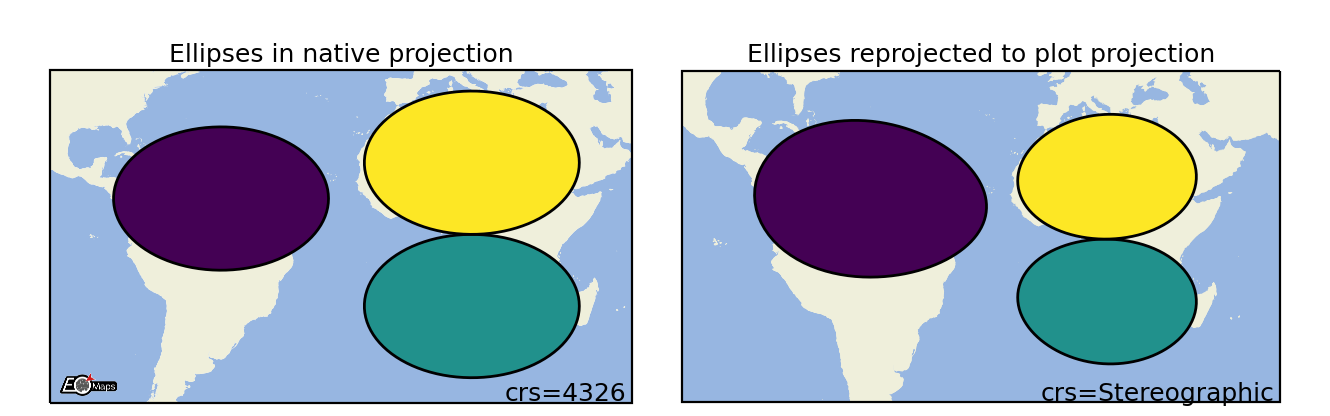Suitable data size

Supported data structures

up to ~500k datapoints

1D, 2D or mixed

 `ellipses` Draw projected ellipses with dimensions defined in units of a given crs.
```m.set_shape.ellipses(radius=(2, 5),   # ellipse dimensions [rx , ry]
radius_crs=4326, # projection in which the ellipse is defined
n=50             # number of calculated points on the ellipse
)
```

### Rectangles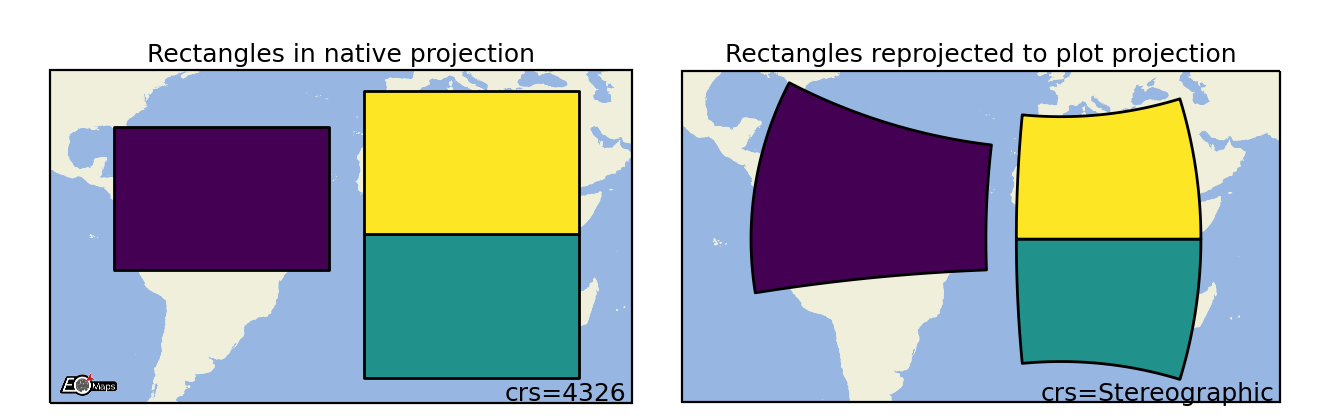Suitable data size

Supported data structures

up to ~500k datapoints

1D, 2D or mixed

 `rectangles` Draw projected rectangles with fixed dimensions (and possibly curved edges).
```m.set_shape.rectangles(radius=(2, 5),   # rectangle dimensions [rx , ry]
radius_crs=4326, # projection in which the rectangle is defined
n=50             # number of calculated points on the ellipse
)
```

### Geodesic Circles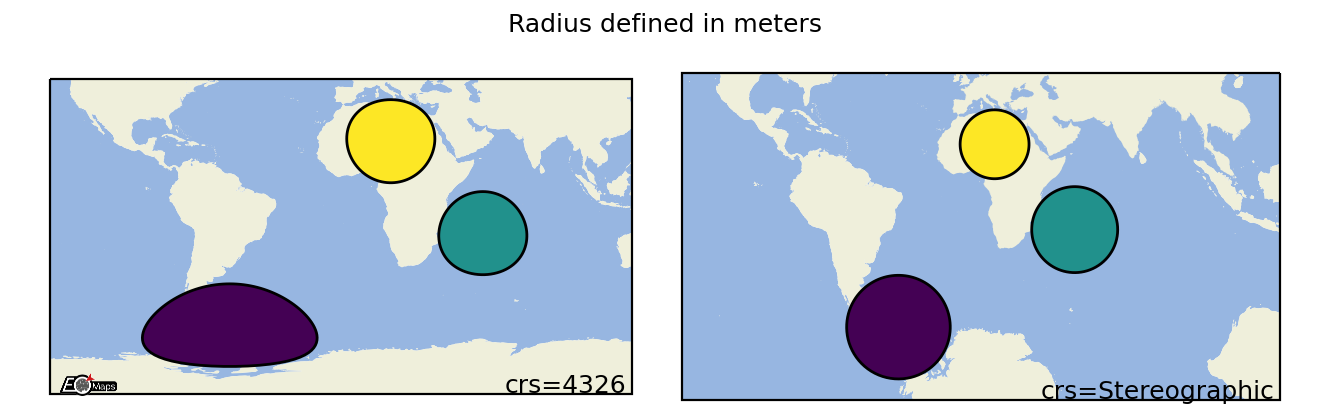Suitable data size

Supported data structures

up to ~500k

1D, 2D or mixed

 `geod_circles` Draw geodesic circles with a radius defined in meters.
```m.set_shape.geod_circles(radius=(2, 5),  # radius in meters
n=50             # number of calculated points on the circle
)
```

### Voronoi Diagram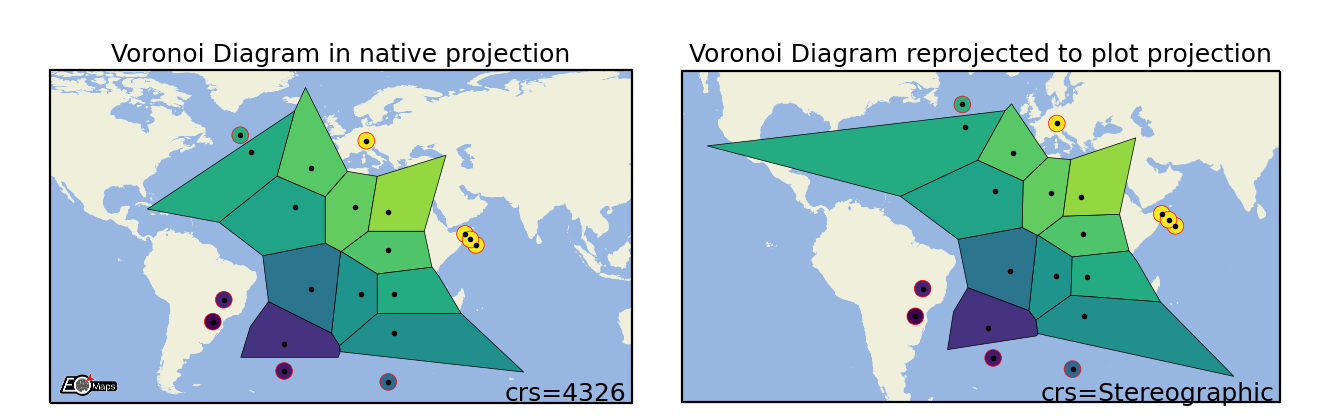Suitable data size

Supported data structures

up to ~500k datapoints

1D, 2D or mixed

 `voronoi_diagram` Draw a Voronoi-Diagram of the data.
```m.set_shape.voronoi_diagram(masked=True,      # mask too large polygons
)
```

### Delaunay Triangulation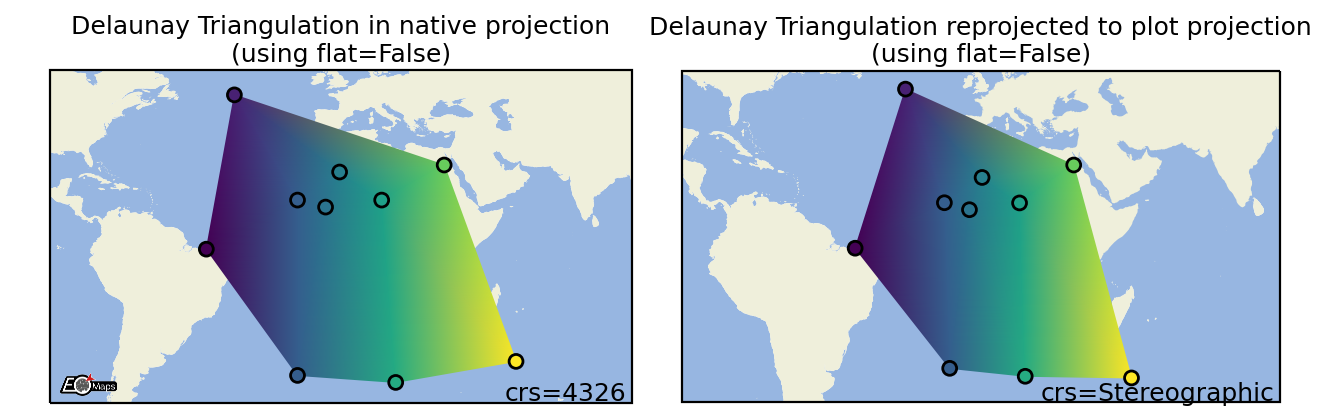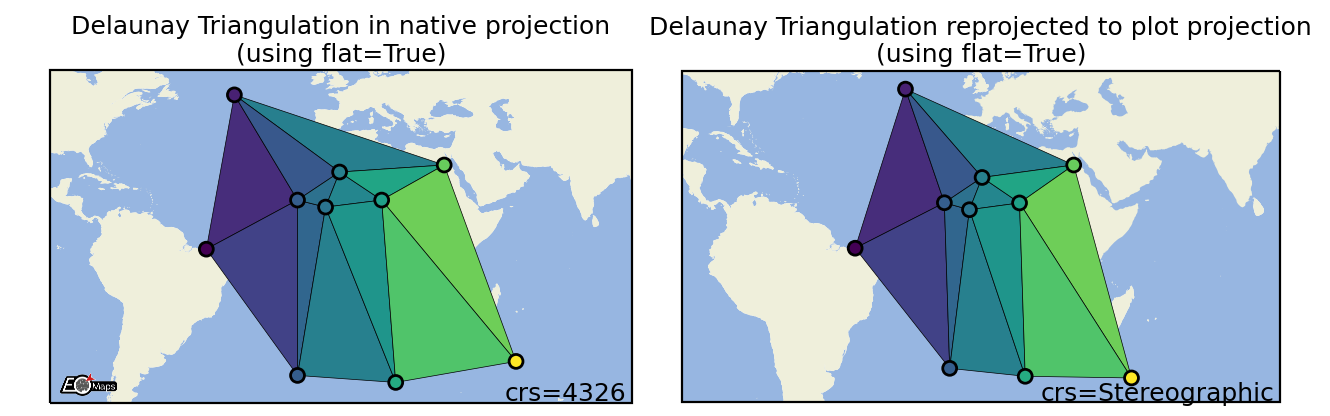Suitable data size

Supported data structures

up to ~500k datapoints

1D, 2D or mixed

 `voronoi_diagram` Draw a Voronoi-Diagram of the data.
```m.set_shape.delaunay_triangulation(flat=False,       # color=mean of triplet (True) or interpolated values (False)
)
```

### Contour plots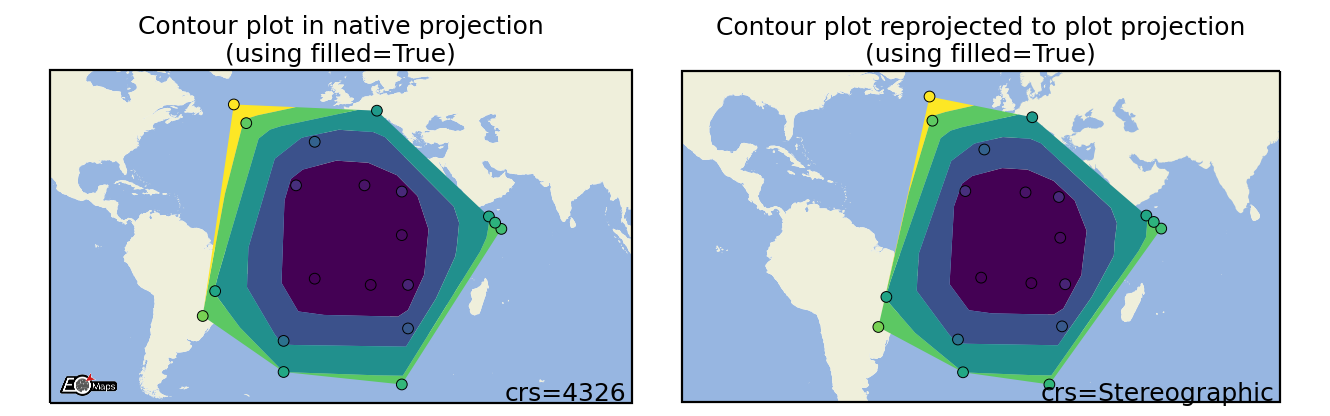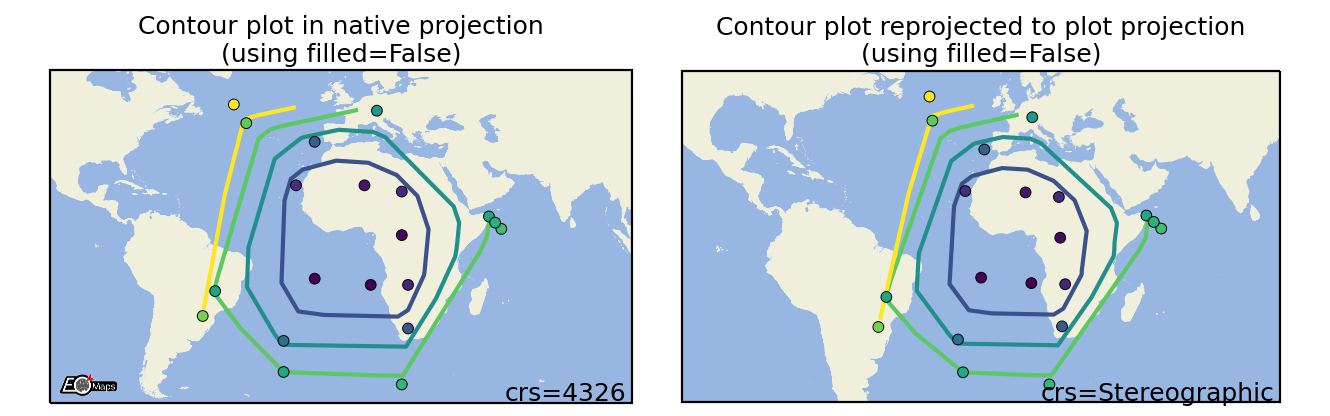Suitable data size

Supported data structures

up to a few million datapoints

1D, 2D or mixed

 `contour` Draw a contour-plot of the data.
```m.set_shape.contour(filled=True)   # filled contour polygons (True) or contour lines (False)
```

### Scatter Points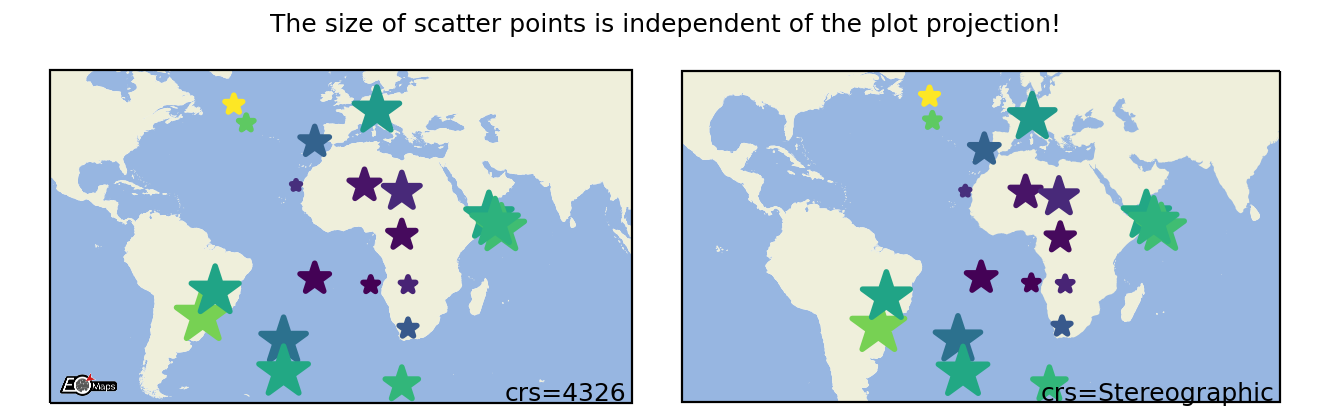Suitable data size

Supported data structures

~500k datapoints

1D, 2D or mixed

 `scatter_points` Draw each datapoint as a shape with a size defined in points**2.
```m.set_shape.scatter_points(size=[1, 2, 3],   # the marker size in points**2
marker="*",       # the marker shape to use
)
```

### Raster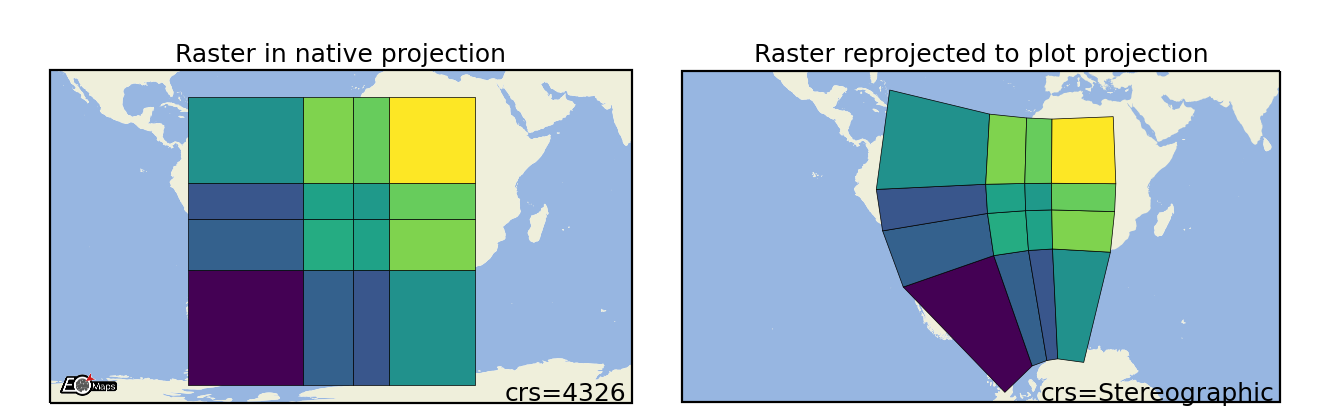Suitable data size

Supported data structures

billions of datapoints (large datasets are pre-aggregated)

2D or 1D coordinates + 2D data

 `raster` Draw the data as a rectangular raster (>> usable for very large datasets!)
```m.set_shape.raster(maxsize=5e5,         # data size at which aggregation kicks in
aggregator='mean',   # aggregation method to use
valid_fraction=0.5,  # % of masked values in aggregation bin for masked result
interp_order=0,      # spline interpolation order for "spline" aggregator
```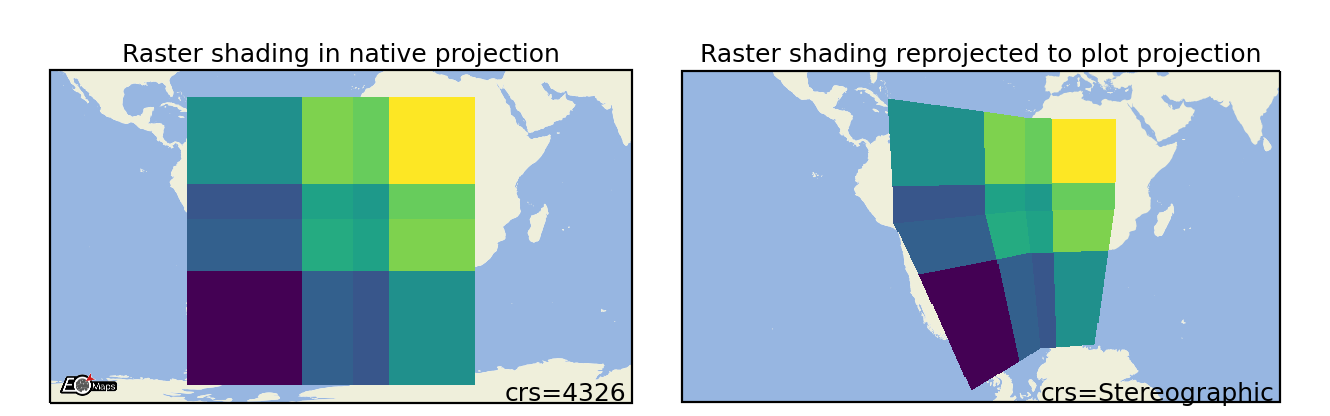Suitable data size

Supported data structures

Optional dependencies

billions of datapoints (large datasets are pre-aggregated)

2D or 1D coordinates + 2D data

 `shade_raster` Shade the data as a rectangular raster (>> usable for very large datasets!).
```m.set_shape.shade_raster(aggregator='mean',    # aggregation method
agg_hook=None,        # datashader aggregation hook callback
)
```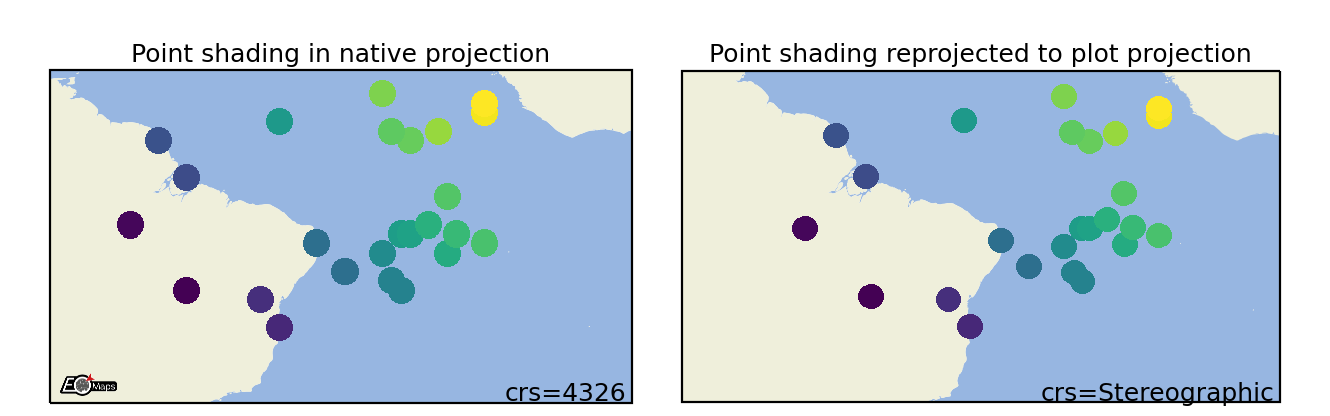Suitable data size

Supported data structures

Optional dependencies

no limit (large datasets are pre-aggregated)

1D, 2D or mixed

 `shade_raster` Shade the data as a rectangular raster (>> usable for very large datasets!).
```m.set_shape.shade_raster(aggregator='mean',    # aggregation method
agg_hook=None,        # datashader aggregation hook callback
)
```

## 1) Classify the data

EOmaps provides an interface for mapclassify to classify datasets prior to plotting.

To assign a classification scheme to a `Maps` object, use `m.set_classify.< SCHEME >(...)`.

• Available classifier names are accessible via `Maps.CLASSIFIERS`.

 `Maps.set_classify` Interface to the classifiers provided by the 'mapclassify' module.
 ```from eomaps import Maps import numpy as np data = np.random.normal(0, 1, (50, 50)) x = np.linspace(-45, 45, 50) y = np.linspace(-45, 45, 50) m = Maps(figsize=(4, 5)) m.add_feature.preset.coastline(lw=2) m.add_feature.preset.ocean(zorder=99, alpha=0.5) m.set_data(data, x, y) m.set_shape.ellipses() m.set_classify.StdMean(multiples=[-1.5, -.5, .5, 1.5]) m.plot_map(vmin=-3, vmax=3) cb = m.add_colorbar(pos=0.2, label="StdMean classification") cb.tick_params(labelsize=7) ```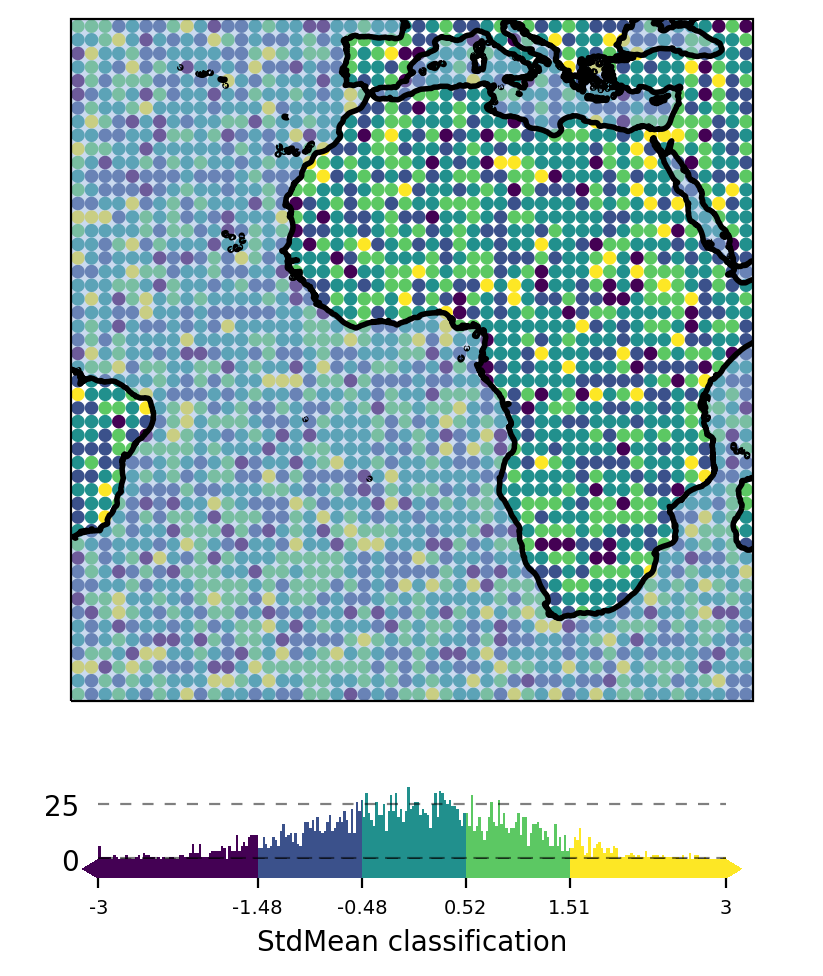Currently available classification-schemes are (see mapclassify for details):

## 4) Plot the data

Now that the data is assigned to a `Maps` object, you can trigger plotting the data via `Maps.plot_map()`.

Any additional keyword-arguments passed to `Maps.plot_map()` are forwarded to the actual `matplotlib` plot-command for the selected shape.

Useful arguments that are supported by all shapes are:

• `"cmap"` : the colormap to use

• `"vmin"`, “vmax” : the range of values used when assigning the colors

• `"alpha"` : the color transparency

• `"zorder"` : the “stacking-order” of the feature

Arguments that are supported by all shapes except `shade` shapes are:
• `"fc"` or `"facecolor"` : set the face color for the whole dataset

• `"ec"` or `"edgecolor"` : set the edge color for the whole dataset

• `"lw"` or `"linewidth"` : the line width of the shapes

By default, the plot-extent of the axis is adjusted to the extent of the data if the extent has not been set explicitly before. To always keep the extent as-is, use `m.plot_map(set_extent=False)`.

```from eomaps import Maps
m = Maps()

m.set_data([1,2,3,4,5], [10,20,40,60,70], [10,20,50,70,30], crs=4326)
m.plot_map(cmap="viridis", ec="b", lw=1.5, alpha=0.85, set_extent=False)
```

You can then continue to add a 🌈 Colorbars (with a histogram) or create Zoomed in views on datasets.

 `Maps.plot_map` Plot the dataset assigned to this Maps-object. `Maps.savefig` Save the current figure.

## Customize the plot

All arguments to customize the appearance of a dataset are passed to `Maps.plot_map()`.

In general, the colors assigned to the shapes are specified by

• selecting a colormap (`cmap`)

• either a name of a pre-defined `matplotlib` colormap (e.g. `"viridis"`, `"RdYlBu"` etc.)

• or a general `matplotlib` colormap object (see matplotlib-docs for more details)

• (optionally) setting appropriate data-limits via `vmin` and `vmax`.

• `vmin` and `vmax` set the range of data-values that are mapped to the colorbar-colors

• Any values outside this range will get the colormaps `over` and `under` colors assigned.

```from eomaps import Maps
m = Maps()
m.set_data([1,2,3,4,5], [10,20,40,60,70], [10,20,50,70,30])
m.plot_map(cmap="viridis", vmin=2, vmax=4, ec="b", lw=0.5)
```

Colors can also be set manually by providing one of the following arguments to `Maps.plot_map()`:

• to set both facecolor AND edgecolor use `color=...`

• to set the facecolor use `fc=...` or `facecolor=...`

• to set the edgecolor use `ec=...` or `edgecolor=...`

Note

• Manual color specifications do not work with the `shade_raster` and `shade_points` shapes!

• Providing manual colors will override the colors assigned by the `cmap`!

• The `colorbar` does not represent manually defined colors!

### Uniform colors

To apply a uniform color to all datapoints, you can use matpltolib’s named colors or pass an RGB or RGBA tuple.

• `m.plot_map(fc="orange")`

• `m.plot_map(fc=(0.4, 0.3, 0.2))`

• `m.plot_map(fc=(1, 0, 0.2, 0.5))`

```from eomaps import Maps

m = Maps()
m.set_data(data=None, x=[10,20,30], y=[10,20,30])

# Use any of matplotlibs "named colors"
m1 = m.new_layer(inherit_data=True)
m1.plot_map(fc="r", zorder=0)

m2 = m.new_layer(inherit_data=True)
m2.plot_map(fc="orange", zorder=1)

# Use RGB or RGBA tuples
m3 = m.new_layer(inherit_data=True)
m3.plot_map(fc=(1, 0, 0.5), zorder=2)

m4 = m.new_layer(inherit_data=True)
m4.plot_map(fc=(1, 1, 1, .75), zorder=3)

# For grayscale use a string of a number between 0 and 1
m5 = m.new_layer(inherit_data=True)
m5.plot_map(fc="0.3", zorder=4)
```

### Explicit colors

To explicitly color each datapoint with a pre-defined color, simply provide a list or array of the aforementioned types.

```from eomaps import Maps

m = Maps()
m.set_data(data=None, x=[10, 20, 30], y=[10, 20, 30])

# Use any of matplotlibs "named colors"
# (https://matplotlib.org/stable/gallery/color/named_colors.html)
m1 = m.new_layer(inherit_data=True)
m1.plot_map(fc=["indigo", "g", "orange"], zorder=1)

# Use RGB tuples
m2 = m.new_layer(inherit_data=True)
m2.plot_map(fc=[(1, 0, 0.5),
(0.3, 0.4, 0.5),
(1, 1, 0)], zorder=2)

# Use RGBA tuples
m3 = m.new_layer(inherit_data=True)
m3.plot_map(fc=[(1, 0, 0.5, 0.25),
(1, 0, 0.5, 0.75),
(0.1, 0.2, 0.5, 0.5)], zorder=3)

# For grayscale use a string of a number between 0 and 1
m4 = m.new_layer(inherit_data=True)
m4.plot_map(fc=[".1", ".2", "0.3"], zorder=4)
```

### RGB/RGBA composites

To create an RGB or RGBA composite from 3 (or 4) datasets, pass the datasets as tuple:

• the datasets must have the same size as the coordinate arrays!

• the datasets must be scaled between 0 and 1

If you pass a tuple of 3 or 4 arrays, they will be used to set the RGB (or RGBA) colors of the shapes, e.g.:

• `m.plot_map(fc=(<R-array>, <G-array>, <B-array>))`

• `m.plot_map(fc=(<R-array>, <G-array>, <B-array>, <A-array>))`

You can fix individual color channels by passing a list with 1 element, e.g.:

• `m.plot_map(fc=(<R-array>, [0.12345], <B-array>, <A-array>))`

```from eomaps import Maps
import numpy as np

x, y = np.meshgrid(np.linspace(-20, 40, 100),
np.linspace(50, 70, 50))

# values must be between 0 and 1
r = np.random.randint(0, 100, x.shape) / 100
g = np.random.randint(0, 100, x.shape) / 100
b = [0.4]
a = np.random.randint(0, 100, x.shape) / 100

m = Maps()
m.set_data(data=None, x=x, y=y)
m.plot_map(fc=(r, g, b, a))
```

## 🌈 Colorbars (with a histogram)

Before adding a colorbar, you must plot the data using `m.plot_map(vmin=..., vmax=...)`.

• `vmin` and `vmax` hereby specify the value-range used for assigning colors (e.g. the limits of the colorbar).

• If no explicit limits are provided, the min/max values of the data are used.

• For more details, see 🔴 Data Visualization.

Once a dataset has been plotted, a colorbar with a colored histogram on top can be added to the map by calling `Maps.add_colorbar()`.

Note

The colorbar always represents the dataset that was used in the last call to `Maps.plot_map()`.
If you need multiple colorbars, use an individual `Maps` object for each dataset! (e.g. via `m2  = m.new_layer()`)

Note

Colorbars are only visible if the layer at which the data was plotted is visible!

 ```from eomaps import Maps import numpy as np data = np.random.normal(0, 1, (50, 50)) x = np.linspace(-45, 45, 50) y = np.linspace(-45, 45, 50) m = Maps(layer="all") m.add_feature.preset.coastline() m.add_feature.preset.ocean(zorder=99, alpha=0.5) m.util.layer_selector(loc="upper left") mA = m.new_layer("A") mA.set_data(data, x, y) mA.set_classify.Quantiles(k=5) mA.plot_map(vmin=-3, vmax=3) cbA = mA.add_colorbar(label="Quantile classification") cbA.tick_params(rotation=45) mB = m.new_layer("B") mB.set_data(data, x, y) mB.set_classify.EqualInterval(k=5) mB.plot_map(vmin=-3, vmax=3) cbB = mB.add_colorbar(label="EqualInterval classification") cbB.tick_params(labelcolor="darkblue", labelsize=9) m.subplots_adjust(bottom=0.1) mA.show() ```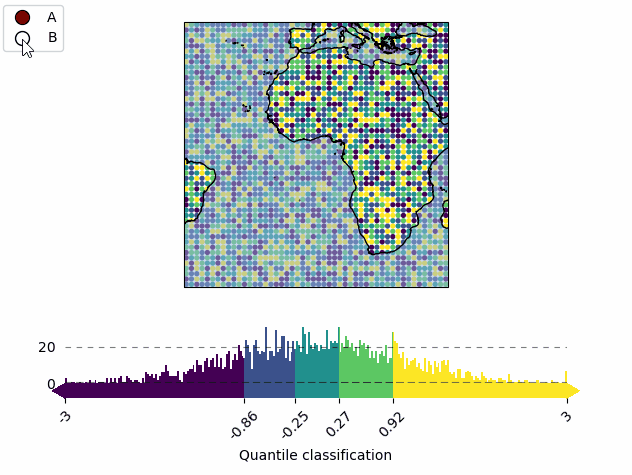`Maps.add_colorbar` Add a colorbar to the map.

The returned `ColorBar`-object has the following useful methods defined:

 `ColorBar.set_position` Set the position of the colorbar (and all colorbars that share the same location) `ColorBar.set_labels` Set the labels (and the styling) for the colorbar (and the histogram). `ColorBar.set_hist_size` Set the size of the histogram (relative to the total colorbar size) `ColorBar.tick_params` Set the appearance of the colorbar (or histogram) ticks. `ColorBar.set_visible` Set the visibility of the colorbar. `ColorBar.remove` Remove the colorbar from the map.

### Set colorbar tick labels based on bins

To label the colorbar with custom names for a given set of bins, use `ColorBar.set_bin_labels()`:

 ```import numpy as np from eomaps import Maps # specify some random data lon, lat = np.mgrid[-45:45, -45:45] data = np.random.normal(0, 50, lon.shape) # use a custom set of bins to classify the data bins = np.array([-50, -30, -20, 20, 30, 40, 50]) names = np.array(["below -50", "A", "B", "C", "D", "E", "F", "above 50"]) m = Maps() m.add_feature.preset.coastline() m.set_data(data, lon, lat) m.set_classify.UserDefined(bins=bins) m.plot_map(cmap="tab10") m.add_colorbar() # set custom colorbar-ticks based on the bins m.colorbar.set_bin_labels(bins, names) ```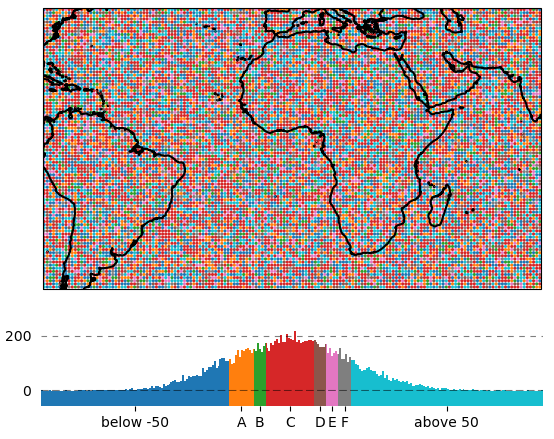`ColorBar.set_bin_labels` Set the tick-labels of the colorbar to custom names with respect to a given set of bins.

### Using the colorbar as a “dynamic shade indicator”

Note

This will only work if you use `m.set_shape.shade_raster()` or `m.set_shape.shade_points()` as plot-shape!

For shade shapes, the colorbar can be used to indicate the distribution of the shaded pixels within the current field of view by setting `dynamic_shade_indicator=True`.

 ```from eomaps import Maps import numpy as np x, y = np.mgrid[-45:45, 20:60] m = Maps() m.add_feature.preset.coastline() m.set_data(data=x+y, x=x, y=y, crs=4326) m.set_shape.shade_raster() m.plot_map() m.add_colorbar(dynamic_shade_indicator=True, hist_bins=20) ```